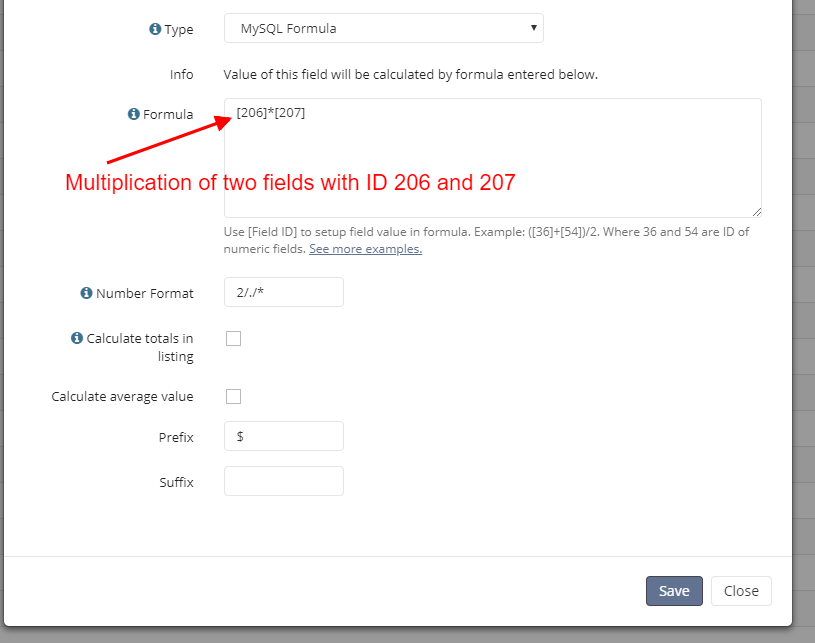To work with mathematical calculations in program Rukovoditel there are two types of fields: "MySql Formula" and "JS Formula". By analogy with the Excel table cell, this field displays the result of the specified arithmetic operations. When you create such a field, you specify the fields whose values you want to use and the calculations that you want to perform.In MySql formula field type the mathematical calculations occur after entering data into the database. This formula is built into the MySql query, which gives you the ability to use any mathematical MySql functions for calculations, as well as other MySql functions and operators. Also, you can apply functions from the Extension to perform calculations from other database tables.

# Simple calculations

`(+)/2`

where 36 and 54 — id of numeric fields.

Mathematical functions

In the formula we can apply a mathematical function of MySql. For example, we need to round the calculation result to an integer:

`FLOOR((+)/2)`

The calculation formula with the condition

To add a condition in the formula we need to use an IF statement.

`if(expr1,expr2,expr3)`

If `expr1` is `TRUE` (`expr1 <> 0` and `expr1 <> NULL`) then `IF()` returns `expr2`; otherwise it returns `expr3``IF()` returns a numeric or string value, depending on the context in which it is used.

`if(>0,(+)/2,0)`

In this example, if the value 36 is greater than 0, then we do the calculation, otherwise, return zero.

If you  need to return a string, such as a blank, or the entered text, then the string must be enclosed in single quotation marks, for example:

`if(>0,(+)/2,'no data')`

Check the list choices  in the IF statement

It is very often necessary to make calculations only in the case if, for example the field “Project Status” has field type “Dropdown” and value “Completed”. In this case, the condition will be as follows:

`if(=15,(+)/2,0)`

where 11 is the ID for the Status field, and 15 is the ID of the value “Completed”.

Now let’s look at an example where we need to check multiple values of statues:

`if( in (15,16,17),(+)/2,0)`

where 15,16,17 are the ids of the choices values.

To check the value of the Boolean Field, use ‘true’ or ‘false’ example:

```if(='true',(+)/2,0)

```

Check the list choices with `CASE`

The CASE statement is an analog of the switch statement in PHP. Its construction is as follows:

```case
when  =15 then (+)/2)
when  =16 then (+)/3)
end```

# The calculation of the time difference

Very often there is a need to create a calculation of time difference between Start Date and End Date of the Project or Tasks.

Dates are stored in a database in seconds, then calculate the number of days will be the following:

`(-)/86400`

where 300 is the ID of the field “End Date”, 159 — ID of the field “Start Date”, 86400 — the number of seconds in a day.

But as the date can be entered incorrectly or be missing altogether, then we need to do checks before the calculation. The correct formula would be as follows:

`if(>0 and >0 and >,(-)/86400,0)`

Note: you can use [TODAY] for current date.

Output date format using FROM_UNIXTIME

`FROM_UNIXTIME(,'%Y-%m-%d')`

where 12 is the identifier for the date field

Date and Time Functions

In MySQL formula field type, you can perform calculations using data and time functions. Example:

`DAYOFMONTH(FROM_UNIXTIME(,'%Y-%m-%d'))`

Merge several fields

Using the CONCAT function, you can merge several fields into a string.

```CONCAT(,' - ',,' - ',id)
```

# MySQL query

You can embed your own MySQL query in the MySQL formula field. For example, the following query displays the email address of the user who created the record.

`SELECT app_entity_1.field_9 FROM app_entity_1 WHERE app_entity_1.id = e.created_by`

Internal variable

`[TODAY]` - current date in UNIXTIME.
`[id]` - current item id.
`[date_added]` - date added in UNIXTIME.
`[created_by]` - id of the user who created the record.
`[parent_item_id]` - parent item id.
`[current_user_id]` - current user id.

# get_value()

For fields of type List, you can set the values of each option, and with internal function get_value () you can use these values in the formula fields.

`++get_value()`

where 15 is the identifier of a field of type List. If field 15 is not mandatory and can be empty, in mathematical expressions it is necessary to check the NULL result.

```++IFNULL(get_value(),0)
```

# parent_entity_item_value()

Use this function to get value from parent entity item. You can get value from any parents in entities tree.

`parent_entity_item_value(22,173)`

where 22 - is parent entity ID, 173 - is field ID in parent entity.

Note: You can select any fields that stored in database. You can't select dynamic fields in this way.

Also this function create several nested sql queries which puts a load on the server. If you need get several values from parent item, better store them in "static text pattern" field and select just one field than to use the parent_entity_item_value () function multiple times.

# entity_item_value()New feature in 3.0

Use this function to get the value from the related entity by the "Entity" field.

`entity_item_value(13,17)`

13 - the type of the entity field, 17-the id of the field whose value should be used in the formula.

Note: You can select any fields that stored in database. You can't select dynamic fields in this way.

# Functions

Functions help you to perform calculations using fields from other entities. To add a function in a formula, use brackets. Consider a simple example of using functions:

`{12}+`

where 12 is the ID function and 5 the ID number field.

The following example will allow you to perform the calculation with several functions:

`{12}+{13}`

Also functions can be applied in conditions:

`if(>0,{12},0)`
```if({1}={2},'<span style="color:green">In Stock</span>','NO')
```

# NULL in response

If there are no records in the entity, the function will return NULL. When working with NULL, there are special rules that do not allow NULL to be used in mathematical expressions. In this regard, it is necessary to use the  IFNULL check:

```IFNULL({12},0)+
```